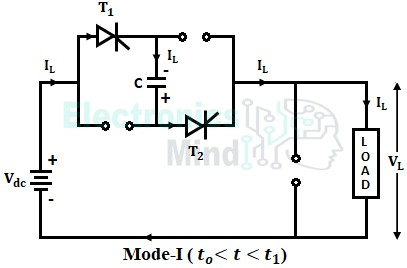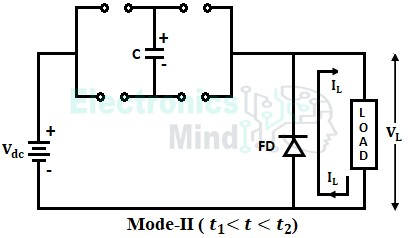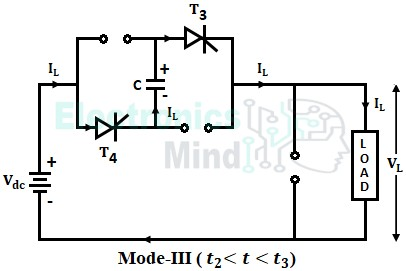The chopper circuit employing the load commutation technique is known as the load commutation chopper. The load commutation technique is, falling of the load current flowing through the thyristor to zero due to the nature of the load itself.

So that the conducting thyristor gets commutated once the current flowing through it falls below the holding current value. Let us see the circuit diagram, operation, and waveforms of the load commutated chopper.

## Circuit Diagram of Load Commutated Chopper :

The circuit diagram of the load commutated chopper is shown below. The circuit consists of four thyristors (T1, T2, T3, and T4) and a commutating capacitor. The four thyristors operate in two pairs, thyristors T1, T2 form one pair, and thyristors T3, T4 form another pair. The two thyristor pairs are triggered alternatively and conduct load current.

In load commutated chopper, at an instant, there are two main thyristors that conduct load current along with the commutating circuit. Whenever thyristors pair T1, T2 are acting as main thyristors, the thyristors pair T3, T4 along with the capacitor form the commutating circuit.

Whereas, when thyristors pair T3, T4 are acting as main thyristors, the thyristors pair T1, T2 along with the capacitor form the commutating circuit. A free-wheeling diode is connected across the load to free-wheel the inductive load current back to it.

## Working of Load Commutated Chopper :

Now let us see the operation of the above load commutated chopper. In the above circuit, it should be assumed that the losses in diode and thyristors are zero and the load current is assumed to be constant. The operation of the circuit can be explained in the following modes.

#### Before starting the circuit, the capacitor C is assumed to be charged to the negative source voltage (-Vdc) with the upper plate as negative, and the lower plate as positive as shown below.Mode-I : At instant t = t0, the thyristors pair T1, T2 is triggered. Hence, the load current flows through the path, Vdc+ → T1 → C → T2 → Load → Vdc– as shown below. The load voltage will be equal to VL = (Vdc + VC) = 2Vdc.During this, the capacitor is charged linearly by the constant load current from negative source voltage (-Vdc) to positive source voltage (Vdc). When it is fully charged to Vdc, the thyristor pair T1, T2 are reverse biased. Once the load current falls below the holding current value of T1 and T2, they will be turned OFF which will take place at instant t = t1.Mode-II : When T1 and T2 get turned OFF at t1, the freewheeling diode (FD) is forward-biased. Hence the load current is transferred from T1 and T2 to the freewheeling diode. Therefore, the load current flows through the freewheeling diode from t1 to t2 and the load voltage becomes zero as shown below.Mode-III : The thyristors pair T3, T4 are triggered at instant t2. Hence, the load current flows the path Vdc+ → T4 → C → T3 → Load → Vdc– as shown below.The load voltage will be equal to 2Vdc i.e., VL = Vdc + VC = 2Vdc. At instant t3, the capacitor is charged to -Vdc from Vdc, due to which thyristors T3 and T4 are reverse biased.

The output voltage again becomes zero and the load current is transferred to the freewheeling diode from the conducting SCR’s T3 and T4. The capacitor voltage VC will be equal to -Vdc.

The thyristor pair T1 and T2 are triggered again and the whole modes of operation will repeat again. The waveforms of load voltage, load current, capacitor voltage, and gate signals of thyristors are shown below.